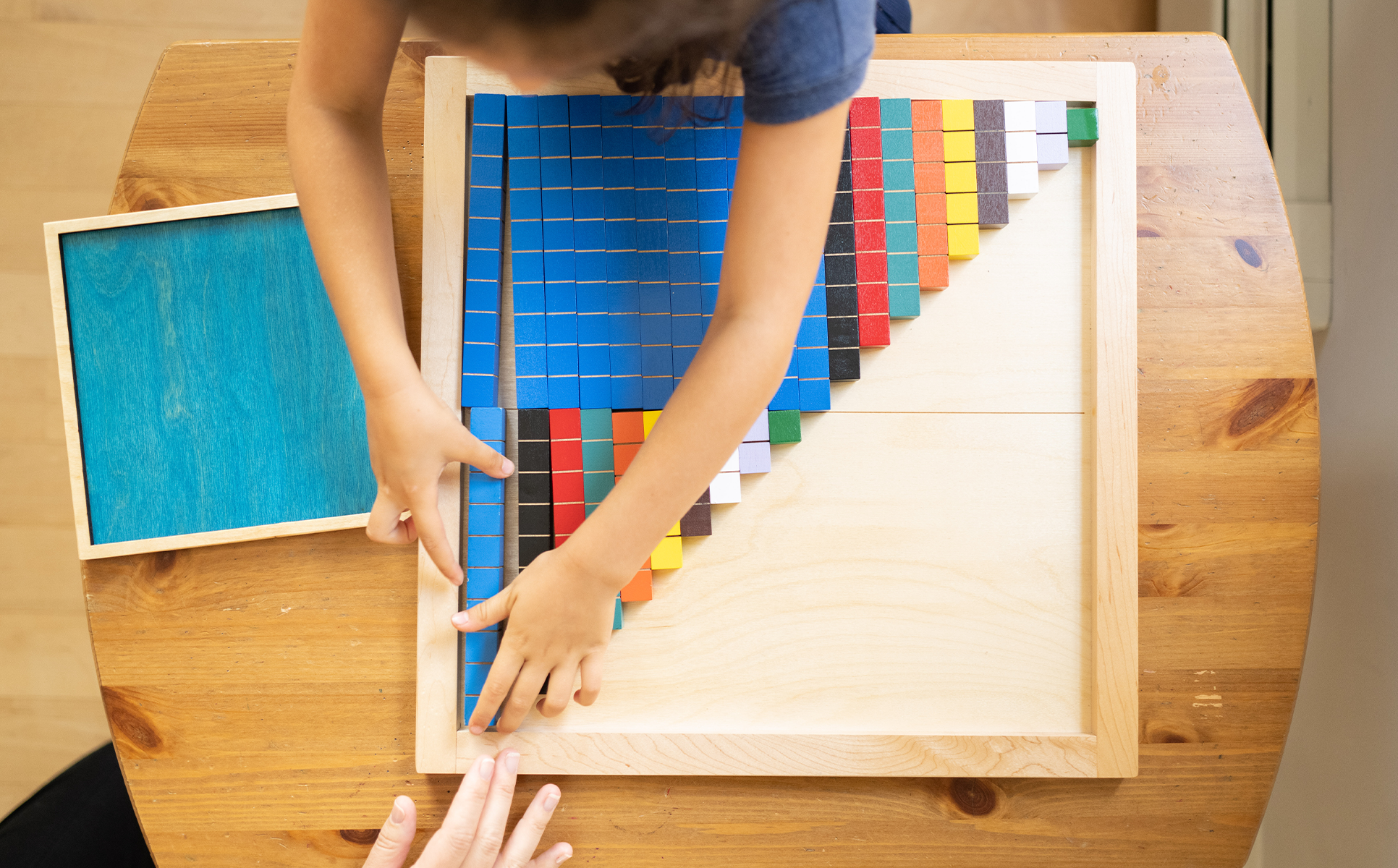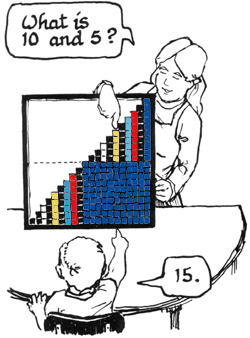# Teaching with the 20 Tray

The 20-Tray demystifies the difficult teen numbers by demonstrating that the sequence and concepts they have internalized regarding numbers 1 to 10 extend to higher numbers, as well. Children discover that the numbers 11-20 are made of ten and some ones, and that the combinations of ten apply to make combinations making twenty e.g. (4+6=10, so 14+6=20).The 20-Tray is used to demonstrate:

• Understanding of teen numbers
• That all teen numbers are made of a ten and some ones
• Counting forwards and backwards within 20
• Fluency with adding 10 and 9 to any number
• Combinations of 20

## Building the 20-Stair

Children discover the role of 10 in each teen number as they build the stair from 1 to 20. They name the blocks in each step, such as 10 and 5, and then give their sum, 15. In this way they all understand the composition of each number from 11 to 20.